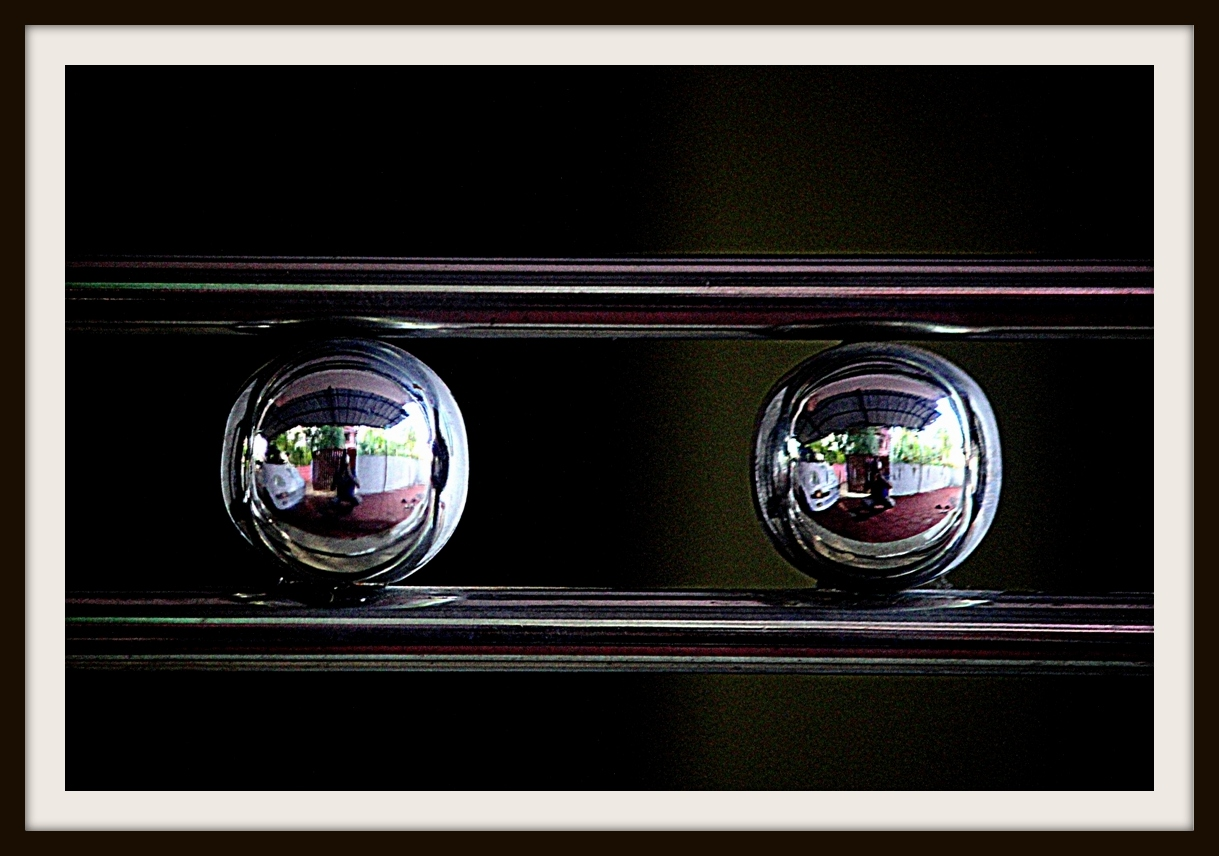# Sphere to cubeA sphere has volume $x \text{ m}^3$ and surface area $x \text{ m}^2$. Keeping its diameter as body diagonal, a cube is made which has volume $a \text{ m}^3$ and surface area $b \text{ m}^2$. What is the ratio $a:b?$

×